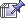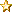October 02, 2023, 05:26:10 pm
 News: Scientists Confirm Historic Massive Flood in Climate Changehttp://www.giss.nasa.gov/research/news/20060228/# The Egyptian Book of the Dead

 Pages: 1 2 3  5 6 7 8 9 10   Go DownAuthor Topic: The Egyptian Book of the Dead  (Read 6018 times)
Josie Linde
Superhero MemberPosts: 4493« Reply #45 on: December 21, 2008, 11:24:40 pm »

List of Chapters.

As in other ancient Theban papyri, this Chapter is without vignette.

Chapter XLIV. "The Chapter of not dying a second time in Neter-khert." (See pp. 105, 315, and pl. 16.)

The vignette is peculiar to the papyrus of Ani.

Chapter XLV. "The Chapter of not suffering corruption in Neter-khert." (See pp. 106, 315, and pl. 16.)

Only one other copy of the text of this Chapter is known. Among Theban papyri the vignette is peculiar to the papyrus of Ani.

Chapter XLVI. "The Chapter of not perishing and of becoming alive in Neter-khert." (See pp. 107, 316, and pl. 16.)

Only one other copy of the text of this Chapter is known (B.M. No. 9900). Among Theban papyri the vignette is peculiar to the papyrus of Ani.

Chapter XLVIII. "Another Chapter of one who cometh forth by day against his foes in Neter-khert." (See pp. 123, 321, and pl. 18.)

Only one other copy of the text of this Chapter is known (B.M. No. 9900). Among Theban papyri the vignette is peculiar to the papyrus of Ani.

Chapter L. "The Chapter of not entering in unto the block." (See pp. 108, 315, and pl. 16.)

The text of this Chapter agrees rather with the second version in the papyrus of Nebseni than with that in B.M. papyrus No. 9964. As the Ani papyrus is of Theban origin this was to be expected.

Chapter LIV. "The Chapter of giving breath in Neter-khert." (See pp. 94, 310, and pl. 15.)

Only one other copy of this Chapter is known, and it is without vignette.

Chapter LVIII. " The Chapter of breathing the air, and of having power over the water in Neter-khert." (See pp. 103, 314, and pl. 16.)

No other copy of this Chapter is known.

Chapter LIX. "The Chapter of breathing the air, and of having power over the water in Neter-khert." (See pp. 104, 315, and pl. 16.)

Only one other copy of this Chapter is known.

Chapter LXI. "The Chapter of not letting the soul of a man be taken away from him in Neter-khert." (See pp. 91, 309, and pl. 15.)

The vignette is similar to that in the papyrus of Sutimes, which M. Naville believes to be no older than the XIXth dynasty.

[1. Naville, Einleitung, p. 134.

2. Ibid., p. 136.

3. Ibid., p. 100.]

{p. cl}

List of Chapters

Chapter LXXII.--Rubric. (See pp. 26, 275, and pl. 6.)

Chapter LXXIV. "The Chapter of walking with the legs and of coming forth upon earth." (See pp. 118, 320, and pl. 18.)

Chapter LXXVII. " The Chapter of changing into a golden hawk ." (See pp. 152, 332, and pl. 25.)

Chapter LXXVIII. "The Chapter of changing into a divine hawk." (See pp. 154, 333, and pl. 25, 26.)

Chapter LXXX. "The Chapter of changing into the god who giveth light in the darkness." (See pp. 182, 341, and pl. 28.)

Chapter LXXXIA. "The Chapter of changing into a lotus." (See pp. 181, 340, and pl. 28.)

The pool of water in the vignette is uncommon.

Chapter LXXXII. "The Chapter of changing into Ptah." (See pp. 170, 337, and pl. 27.)

As in other XVIIIth dynasty papyri, this Chapter has a vignette.

Chapter LXXXIII. "The Chapter of changing into a bennu bird" (phœnix?). (See pp. 176, 339, and pl. 27.)

Like other XVIIIth dynasty papyri, this Chapter lacks the addition which is found in the papyrus of Sutimes.

Chapter LXXXIV. "The Chapter of changing into a heron." (See pp. 178, 339, and pl. 28.)

Chapter LXXXV. " The Chapter of changing into the soul of Tmu." (See pp. 172, 338, and pl. 27.)

The vignette to this Chapter is similar to that of the papyrus of Tura, surnamed Nefer-uben-f, of the XVIIIth dynasty.'

Chapter LXXXVI. "The Chapter of changing into a swallow." (See pp. 250, 331, and pl. 25.)

Chapter LXXXVII. "The Chapter of changing into Seta." (See pp. 169, 337, and pl. 27.)

Chapter LXXXVIII. "The Chapter of changing into a crocodile." (See pp. 170, 337, and pl. 27.)

Chapter LXXXIX. "The Chapter of causing the soul to be united to its body in Neter-khert." (See pp. 112, 318, and pl. 17.)

The two incense burners which stand, one at the head and one at the foot of the bier, are peculiar to the papyrus of Ani.

[1. Naville, Einleitung, p. 97.]

{p. cli}

List of Chapters.

Chapter XCI. "The Chapter of not letting the soul of a man be captive in Neter-khert." (See pp. 114, 319, and pl. 17.)

Chapter XCII. "The Chapter of opening the tomb to the soul and the shadow, of coming forth by day, and of getting power over the legs." (See pp. 115, 319, and pl. 17.)

The vignette of this Chapter is unusual and of great interest, for in it Ani's soul accompanies his shadow.

Chapter XCIII. "The Chapter of not letting a man pass over to the east in Neter-khert." (See pp. 109, 317, and pl. 17-)

The vignette as here given is peculiar to the papyrus of Ani.

Chapter XCIIIA. "Another Chapter." (See pp. 110, 317, and pl. 17.)

Chapter CX. "Here begin the Chapters of the Sekhet-hetepu, and the Chapters of coming forth by day, and of going into and coming out from Neter-khert, and of arriving in the Sekhet-Aanru, and of being in peace in the great city wherein are fresh breezes." (See pp. 236, 362, and pl. 34.)

The text is here incomplete.

Chapter CXXIV. "The Chapter of going unto the divine chiefs of Osiris." (See pp. 146, 330, and pl. 24.)

In the vignette we should expect four, instead of three, gods.

Chapter CXXV. "The Chapter of entering into the Hall of double Right and Truth: a hymn of praise to Osiris." (See pp. 189, 344, and pl. 30.)

The Introduction to this Chapter as found in the papyrus of Ani is not met with elsewhere; the text which usually follows the "Negative Confession" is however omitted. The vignette as here given is peculiar to the papyrus of Ani.

Chapter CXXXII. "The Chapter of making a man to return to see again his home upon earth." (See pp. 121, 321, and pl. 18.)

Chapter CXXX III. "[A Chapter] to be said on the day of the month." (See pp. 138, 327, and pl. 21.)

Chapter CXXXIII.--Rubric. (See pp. 142, 328, and pl. 22.)

Chapter CXXXIV. "A hymn of praise to Ra on the day of the month wherein he saileth in the boat." (See pp. 142, 329, and pl. 22.)

Chapter CXLVI. "The Chapter of renewing the pylons in the House of Osiris which is in the Sekhet-Aanru." (See pp. 63, 295, and pll. 11, 12.)

Chapter CXLVII. "A Chapter to be said when Ani cometh to the first Aril." (See pp. 56, 291, and pll. 11, 12.)

Chapter CXLVIII. Without title. See pp. 239, 366, and pl. 35.)

{p. clii}

List of Chapters.

Chapter CLI. Scene in the mummy chamber. (See pp. 229, 360, and Pll. 33, 34.)

Chapter CLV. "The Chapter of a Tet of gold." (See pp. 225, 357, and pl. 33.)

Chapter CLVI. "The Chapter of a Buckle of carnelian." (See pp. 227, 359, and pl. 33.)

Chapter CLXVI. "The Chapter of the Pillow which is placed under the head." (See pp. 228, 359, and pl. 33.)

Chapter CLXXV. " The Chapter of not dying a second time." (See pp. 184, 341, and pl. 29.)

Only one other much mutilated copy of this most important Chapter is known. In it it is declared that neither men nor gods can conceive what great glory has been laid up for Ani in his existence in the next world, and that his life therein shall be for "millions of millions of years."

Chapter CLXXXV. "A Hymn of Praise to Osiris, the dweller in Amenta, Un-nefer within Abtu (Abydos)." (See pp. 241, 367, and pl. 36.)

Chapter CLXXXVI. "A Hymn of praise to Hathor." (See pp. 242, 368. and pl. 37.)
 Report SpamLogged
 Pages: 1 2 3  5 6 7 8 9 10   Go Up